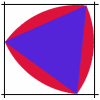#### You may also like### Is There a Theorem?

Draw a square. A second square of the same size slides around the first always maintaining contact and keeping the same orientation. How far does the dot travel?### The Old Goats

A rectangular field has two posts with a ring on top of each post. There are two quarrelsome goats and plenty of ropes which you can tie to their collars. How can you secure them so they can't fight each other but can reach every corner of the field?### Rolling Triangle

The triangle ABC is equilateral. The arc AB has centre C, the arc BC has centre A and the arc CA has centre B. Explain how and why this shape can roll along between two parallel tracks.

# Rollin' Rollin' Rollin'

##### Age 11 to 14Challenge Level

Simon sent us this solution :

The obvious answer is that the outer circle (B say) rotating around the stationary circle (A) revolves once but this is wrong. Looking at the interactivity I could see that the mark on the circumference started on the left of the outer circle and was on the left again when the outer circle had only gone half way around. This means that it must make two full turns as it rotates around the inner circle. Now I need to explain why.

Let's start with each of the circles having a radius r. I thought about the centre of the outer circle. When it makes a full circuit of the inner circle the centre of the outer circle will have drawn a circle of raduis 2r so it will have covered a distance of $2 \pi \times 2r = 4 \pi r$.

So how many rotations has this outer circle made?

I next imagined the circle rolling along a line. How many revolutions would be necessary for the centre to travel the distance of $4\pi r$

In one revolution the centre will travel the same distance as the circle (imagine a bicycle wheel) that is a distance of $2\pi r$. So to travel a distance $4\pi r$ the circle would need to revolve twice. This means the outer circle makes two full turns for every single circuit of the inner circle.

Kevin of Langley Grammer School explained what was happening for circles with different, as well as the same radii.
The centre of the moving circle moves round the circumference of a circle of radius $2r$, i.e. a distance $4\pi r$.

The centre of the moving circle moves a distance $2 \pi r$ when it makes one complete turn about its centre. Therefore when the moving circle returns to $P$ it has made 2 complete turns.

In this situation the ratio of the radii of the moving circle to the non-moving circle was 1.

Let the non-moving circle have a radius of $r$, and the moving circle have a radius of $nr$, so that the ratio of the radii is $n$.

Therefore the centre of the moving circle moves along the circumference of a circle with radius $(1+n)r$, i.e. a distance $2 \pi (1+n)r$. Therefore when the moving circle returns to $P$ it will have made $$\frac{2\pi(1+n)r}{2 \pi nr} = 1+ \frac{1}{n}$$ turns. Therefore if the moving circle has a greater radius than the non-moving circle then $n> 1$, and so $1/n$ would be less than 1, and so the number of turns would be less than 2. If the moving circle has a smaller radius than the non-moving circle then $n< 1$, and so $1/n$ would be greater than 1, meaning that the number of turns would be greater than 2.

It can also be seen that the moving coin makes at least 1 turn, regardless of the sizes of the circles, as 1/n is always positive.

So, if the moving circle is half the radius of the inner circle it will turn $1+2 = 3$ times

If the moving circle is one third the radius of the inner circle - it will turn $1+3 = 4$ times.

Surprised?